# Question 5 [20 marks) Consider Coutte flow, which occurs when we have a fluid suspended between two parallel plates, on...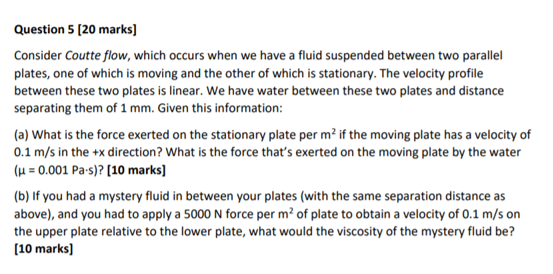Question 5 [20 marks) Consider Coutte flow, which occurs when we have a fluid suspended between two parallel plates, one of which is moving and the other of which is stationary. The velocity profile between these two plates is linear. We have water between these two plates and distance separating them of 1 mm. Given this information: (a) What is the force exerted on the stationary plate per m2 if the moving plate has a velocity of 0.1 m/s in the +x direction? What is the force that's exerted on the moving plate by the water (με 0.001 Pa-s)? [10 marks] (b) If you had a mystery fluid in between your plates (with the same separation distance as above), and you had to apply a 5000 N force per m2 of plate to obtain a velocity of 0.1 m/s on the upper plate relative to the lower plate, what would the viscosity of the mystery fluid be? [10 marks]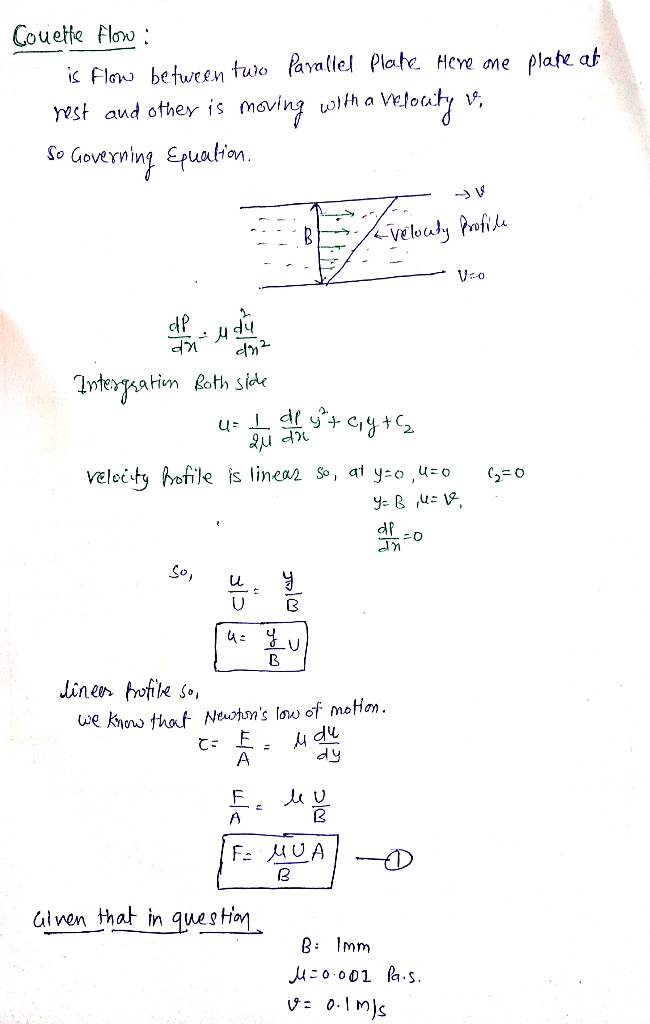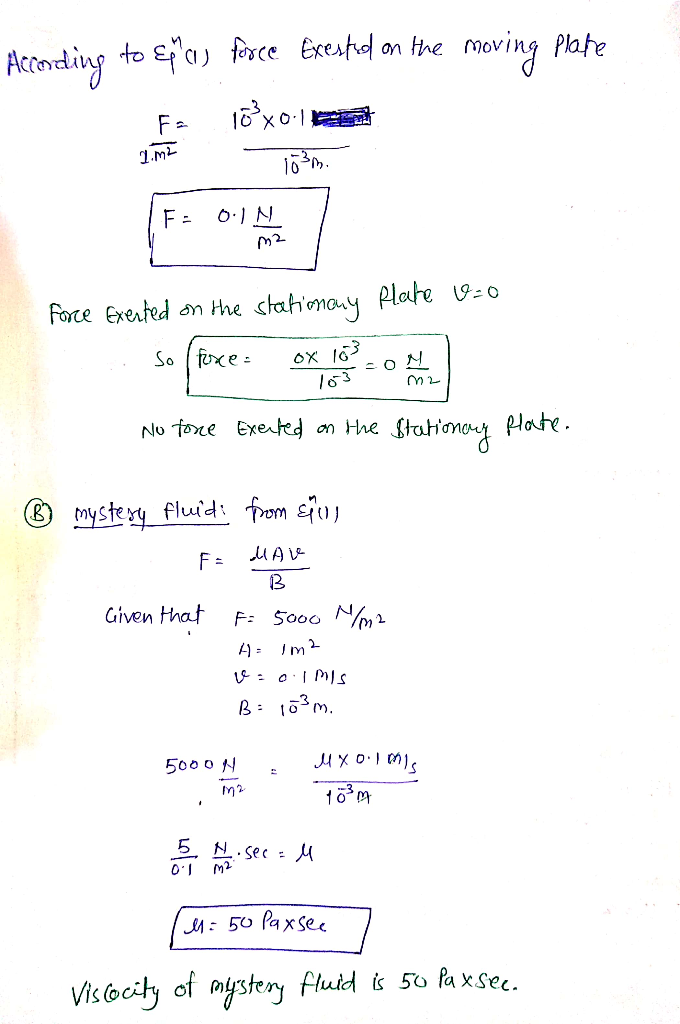##### Add Answer of: Question 5 [20 marks) Consider Coutte flow, which occurs when we have a fluid suspended between two parallel plates, on...
Similar Homework Help Questions
• ### A fluid having a viscosity u 9.35 x 104 lbf-s/ft is contained between two parallel plates,...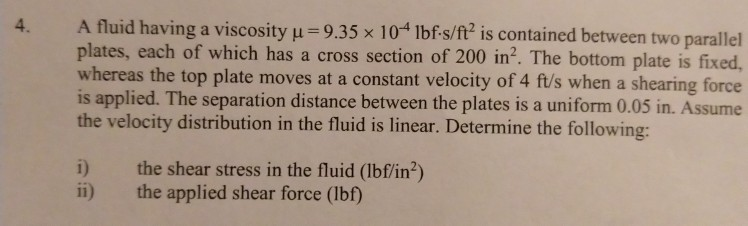A fluid having a viscosity u 9.35 x 104 lbf-s/ft is contained between two parallel plates, each of which has a cross section of 200 in2. The bottom plate is fixed whereas the top plate moves at a constant velocity of 4 ft/s when a shearing force is applied. The separation distance between the plates is a uniform 0.05 in. Assume the velocity distribution in the fluid is linear. Determine the following: 4. the shear stress in the fluid (lbf/in2)...

• ### The velocity distribution for laminar flow between parallel plates is given by where h is the...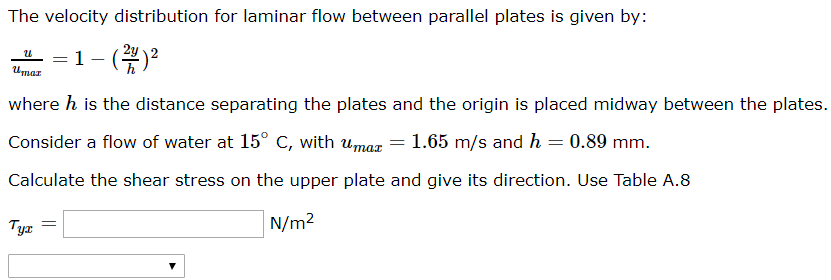The velocity distribution for laminar flow between parallel plates is given by where h is the distance separating the plates and the origin is placed midway between the plates Consider a flow of water at 15° C, with umaz 1.65 m/s and h = 0.89 mm. Calculate the shear stress on the upper plate and give its direction. Use Table A.8 Ti N/m2 yr

• ### Consider steady, incompressible, laminar flow of a Newtonian fluid in the narrow gap between two ...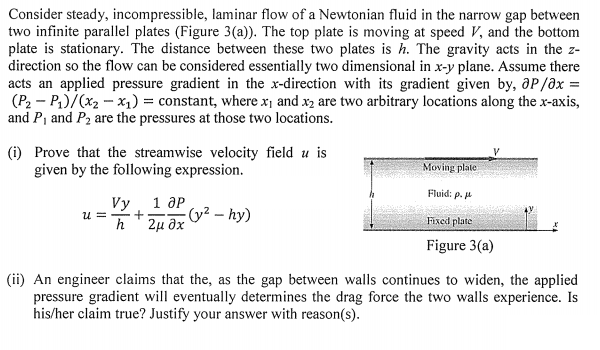Consider steady, incompressible, laminar flow of a Newtonian fluid in the narrow gap between two infinite parallel plates (Figure 3(a)). The top plate is moving at speed V, and the bottom plate is stationary. The distance between these two plates is h. The gravity acts in the z direction so the flow can be considered essentially two dimensional in x-y plane. Assume there acts an applied pressure gradient in the x-direction with its gradient given by, aP/ax (Po-P1)/(x2-x1)-constant, where x1...

• ### Problem 3- For flow of an incompressible, Newtonian fluids between parallel plates, the velocity ...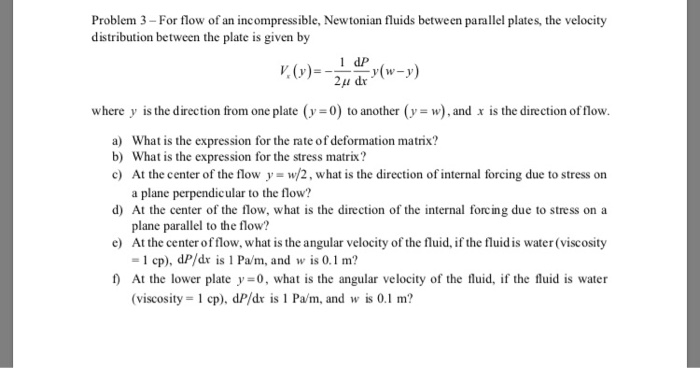Problem 3- For flow of an incompressible, Newtonian fluids between parallel plates, the velocity distribution between the plate is given by 1 dP 2μ dr where y is the direction from one plate (y-0) to another (y-w),and x is the direction of flow a) What is the expression for the rate of deformation matrix? b) What is the expression for the stress matrix? c) At the center of the flow y w/2, what is the direction of internal forcing due...

• ### A thin flat plate is placed in between two parallel plates (top and bottom) as shown...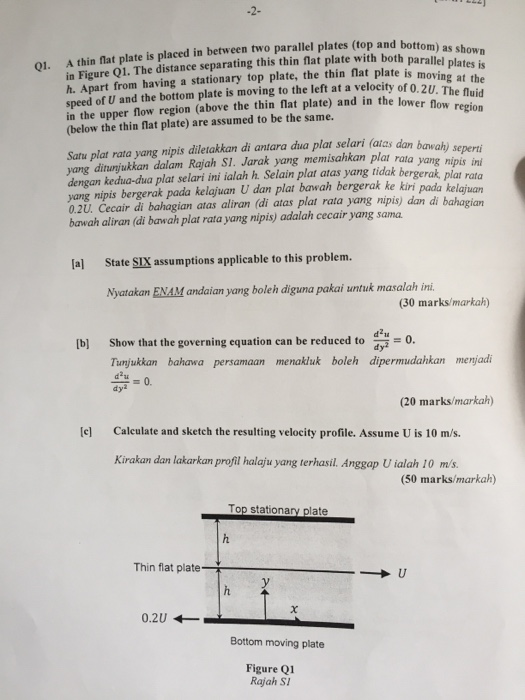A thin flat plate is placed in between two parallel plates (top and bottom) as shown in Figure Q1. The distance separating this thin flat plate with both parallel plates is h. Apart from having a stationary top plate, the thin flat plate is moving at the speed of U and the bottom plate is moving to the left at a velocity of 0.2U. The fluid in the upper flow region (above the thin flat plate) and in the lower...

• ### Two parallel plates, each with zero mass and an area of 10,000 cm2 are set 0.1 mm apart and the space between them is filled with a Newtonian fluid

Two parallel plates, each with zero mass and an area of 10,000 cm2 are set 0.1 mm apart and the space between them is filled with a Newtonian fluid. The upper plate is pushed in the direction shown with a constant force sufficient to accelerate a mass of 1 kg by 1 m/s2, which causes the upper plate to move with a velocity of 1 m/s relative to the lower plate. What is the viscosity of the fluid? Select the...

• ### Q6. marks) Consider an electromagnetic flowmeter which is used to measure the average volumetric flow rate of a pro...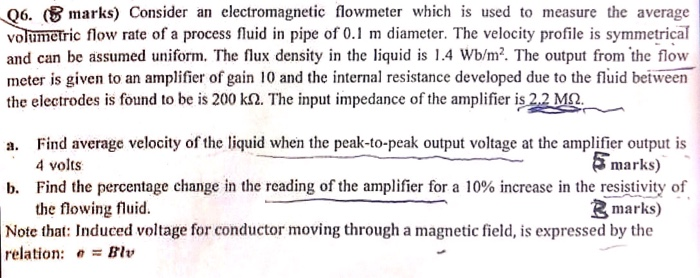Q6. marks) Consider an electromagnetic flowmeter which is used to measure the average volumetric flow rate of a process fluid in pipe of 0.1 m diameter. The velocity profile is symmetrical and can be assumed uniform. The flux density in the liquid is 1.4 Wb/m2. The output from the fow meter is given to an amplifier of gain 10 and the internal resistance developed due to the fluid between the electrodes is found to be is 200 kf2. The input...

• ### 2. Consider a capacitor created when two identical conducting plates are placed parallel and close to...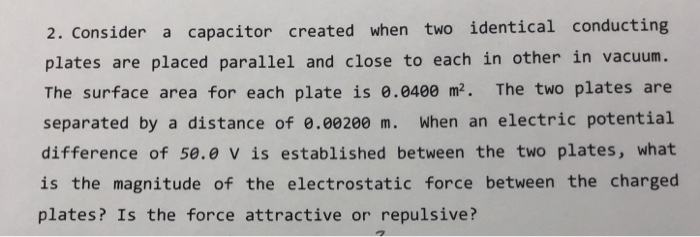2. Consider a capacitor created when two identical conducting plates are placed parallel and close to each in other in vacuum The surface area for each plate is e.0400 m2. The two plates are separated by a distance of e.00200 m. When an electric potential difference of 5e.e v is established between the two plates, what is the magnitude of the electrostatic force between the charged plates? Is the force attractive or repulsive? 3. What mass on the Earth surface...

• ### QUESTION 2A (11 marks) [Allow 13 minutes] Water flows over a stationary, smooth, flat rectangular plate with dimensions 0.4 x 0.25m as shown in the figure below. The plate has its long axis parallel...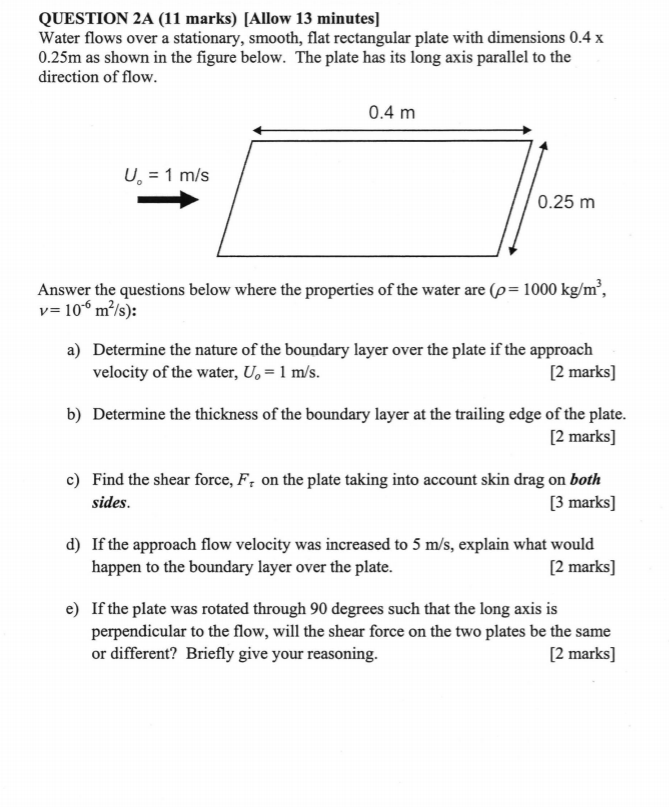QUESTION 2A (11 marks) [Allow 13 minutes] Water flows over a stationary, smooth, flat rectangular plate with dimensions 0.4 x 0.25m as shown in the figure below. The plate has its long axis parallel to the direction of flow 0.4 m U1 m/s 0.25 m Answer the questions below where the properties of the water are (p 1000 kg/m, v 106 m2/s): a) Determine the nature of the boundary layer over the plate if the approach velocity of the water,...

• ### please solve (va20) for me thanks!! :) V VISCOUS FLOWS Page 38 nar flow between two infinite plates a distance h apart driven by a pressure gra- Va20. For lami dient, the velocity profile is [constan...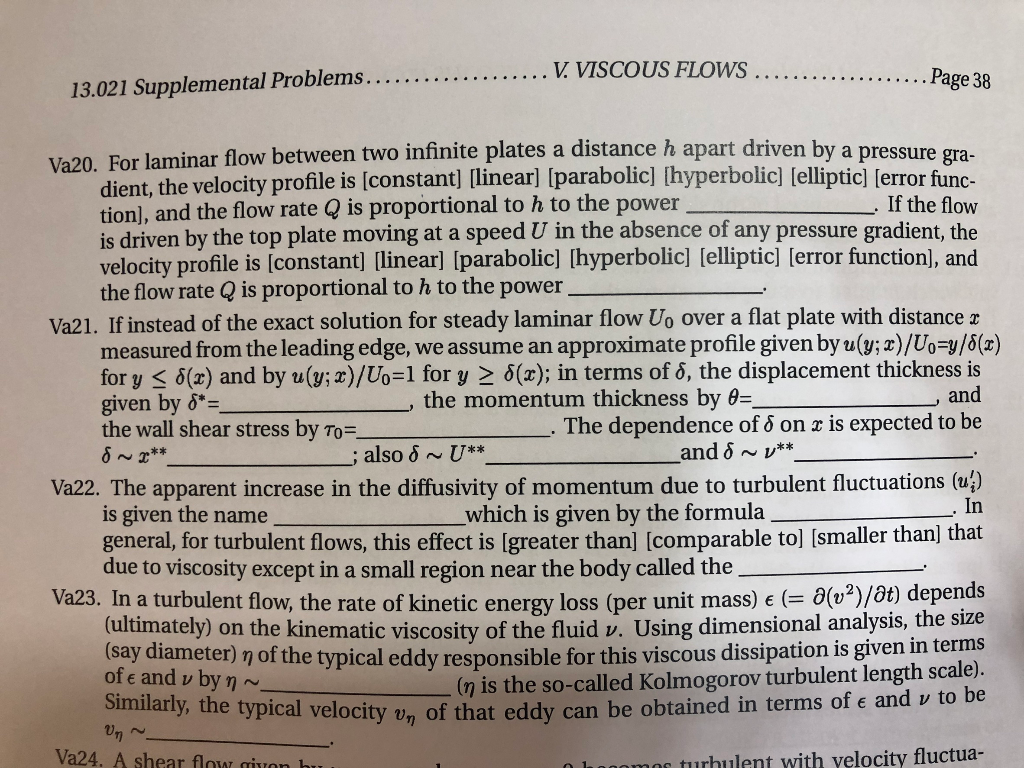please solve (va20) for me thanks!! :) V VISCOUS FLOWS Page 38 nar flow between two infinite plates a distance h apart driven by a pressure gra- Va20. For lami dient, the velocity profile is [constant] [linear] [parabolic] [hyperbolic] [elliptic] [error func- tion], and the flow rate Q is proportional to h to the power is driven by the top plate moving at a speed U in the absence of any pressure gradient, the velocity profile is [constant] linearl Iparabolic]...

Need Online Homework Help?